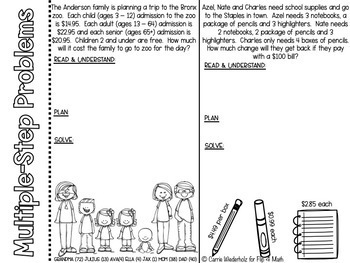# Operations with Decimals Interactive Notebook FoldablesSubject
Resource Type
File Type

PDF

(17 MB|41 pages)
Product Rating
4.0
(2 Ratings)
Standards
• Product Description
• StandardsNEW

This set of interactive notebook foldables on operations with decimals such as:

1. Estimating Sums and Differences of Decimals

3. Estimating Decimal Products and Quotients

4. Multiplying Decimals

5. Dividing Whole Numbers

6. Dividing by a Whole Number

7. Dividing Decimals

8. Evaluating Decimal Expressions (involving PEMDAS)

9. Solutions for Equations and Inequalities (using Substitution)

10. Solving Multiple Step Problems

**BONUS: Dividing Decimals Maze**

All of these foldables are designed to fit in a composition notebook if needed or any other notebook you may use and are designed to be an easy print and go for the teacher. They have a few simple cuts that can be cut by the teacher ahead of time or by the students but nothing that is too time consuming. Many of the foldables have lots of practice which you can use in the classroom for guided practice, independent practice or for homework. Foldables are a great way to help students organize their notes and take pride in their notebook!

Let's Connect

Blog

Instagram

Email: flip4mathteacher@gmail.com

Use variables to represent numbers and write expressions when solving a real-world or mathematical problem; understand that a variable can represent an unknown number, or, depending on the purpose at hand, any number in a specified set.
Understand solving an equation or inequality as a process of answering a question: which values from a specified set, if any, make the equation or inequality true? Use substitution to determine whether a given number in a specified set makes an equation or inequality true.
Evaluate expressions at specific values of their variables. Include expressions that arise from formulas used in real-world problems. Perform arithmetic operations, including those involving whole-number exponents, in the conventional order when there are no parentheses to specify a particular order (Order of Operations). For example, use the formulas V = s³ and A = 6 s² to find the volume and surface area of a cube with sides of length s = 1/2.
Fluently add, subtract, multiply, and divide multi-digit decimals using the standard algorithm for each operation.
Fluently divide multi-digit numbers using the standard algorithm.
Total Pages
41 pages
Included
Teaching Duration
N/A
Report this Resource to TpT
Reported resources will be reviewed by our team. Report this resource to let us know if this resource violates TpT’s content guidelines.